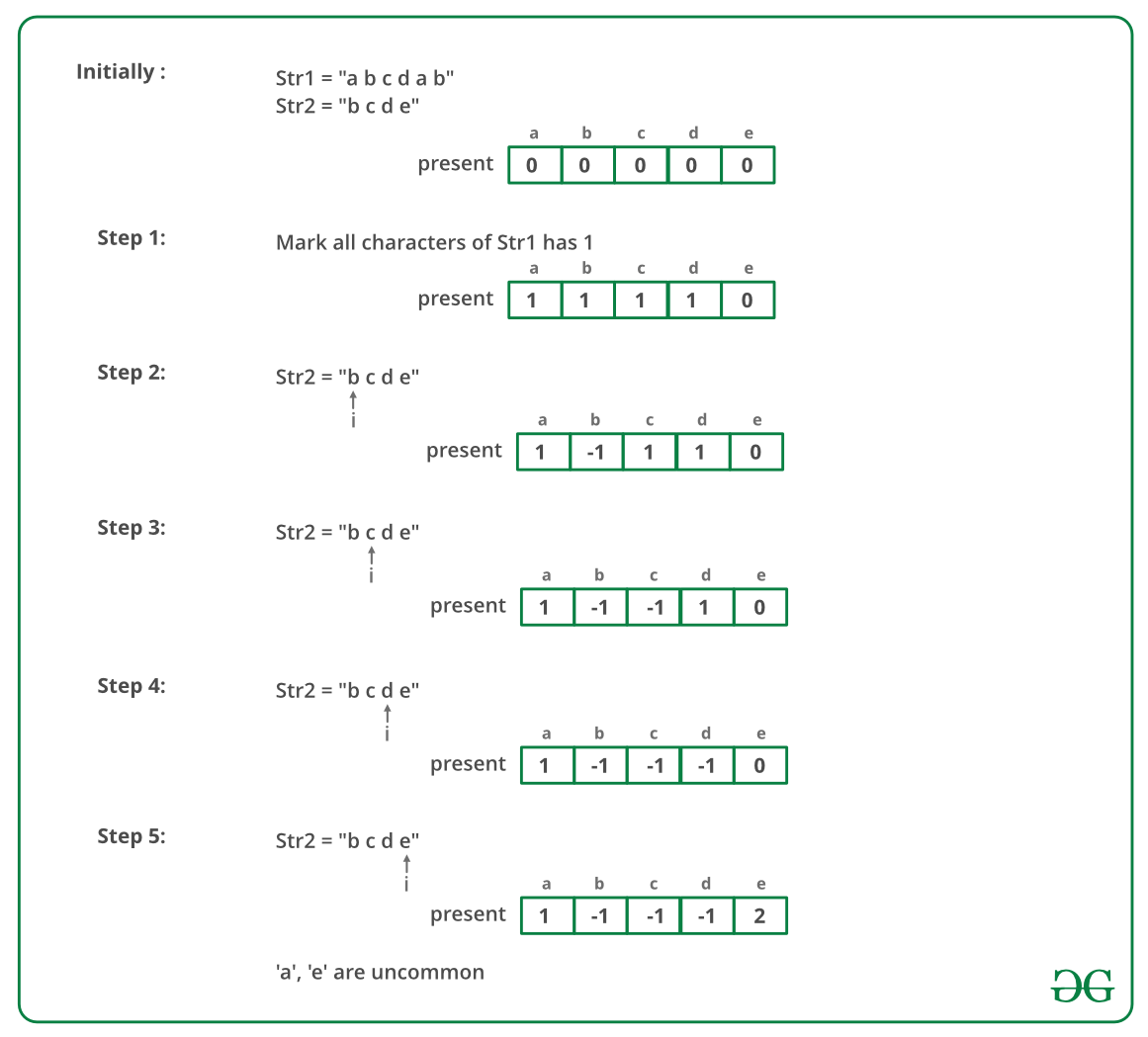Related Articles
Find uncommon characters of the two strings
• Difficulty Level : Easy
• Last Updated : 04 Jul, 2019

Find and print the uncommon characters of the two given strings in sorted order. Here uncommon character means that either the character is present in one string or it is present in other string but not in both. The strings contain only lowercase characters and can contain duplicates.

Examples:

Input: str1 = “characters”, str2 = “alphabets”
Output: b c l p r

Input: str1 = “geeksforgeeks”, str2 = “geeksquiz”
Output: f i o q r u z

## Recommended: Please try your approach on {IDE} first, before moving on to the solution.

Naive Approach: Using two loops, for each character of 1st string check whether it is present in the 2nd string or not. Likewise, for each character of 2nd string check whether it is present in the 1st string or not.

Time Complexity: O(n^2) and extra would be required to handle duplicates.

Efficient Approach: An efficient approach is to use hashing.

• Use a hash table of size 26 for all the lowercase characters.
• Initially, mark presence of each character as ‘0’ (denoting that the character is not present in both the strings).
• Traverse the 1st string and mark presence of each character of 1st string as ‘1’ (denoting 1st string) in the hash table.
• Now, traverse the 2nd string. For each character of 2nd string, check whether its presence in the hash table is ‘1’ or not. If it is ‘1’, then mark its presence as ‘-1’ (denoting that the character is common to both the strings), else mark its presence as ‘2’ (denoting 2nd string).

Below image is a dry run of the above approach:Below is the implementation of the above approach:

## C++

 `// C++ implementation to find the uncommon``// characters of the two strings``#include ``using` `namespace` `std;`` ` `// size of the hash table``const` `int` `MAX_CHAR = 26;`` ` `// function to find the uncommon characters``// of the two strings``void` `findAndPrintUncommonChars(string str1, string str2)``{``    ``// mark presence of each character as 0``    ``// in the hash table 'present[]'``    ``int` `present[MAX_CHAR];``    ``for` `(``int` `i=0; i

## Java

 `// Java implementation to find the uncommon``// characters of the two strings``class` `GFG ``{`` ` `    ``// size of the hash table``    ``static` `int` `MAX_CHAR = ``26``;`` ` `    ``// function to find the uncommon ``    ``// characters of the two strings``    ``static` `void` `findAndPrintUncommonChars(String str1,``                                       ``String str2) ``    ``{``        ``// mark presence of each character as 0``        ``// in the hash table 'present[]'``        ``int` `present[] = ``new` `int``[MAX_CHAR];``        ``for` `(``int` `i = ``0``; i < MAX_CHAR; i++) ``        ``{``            ``present[i] = ``0``;``        ``}`` ` `        ``int` `l1 = str1.length();``        ``int` `l2 = str2.length();`` ` `        ``// for each character of str1, mark its``        ``// presence as 1 in 'present[]'``        ``for` `(``int` `i = ``0``; i < l1; i++)``        ``{``            ``present[str1.charAt(i) - ``'a'``] = ``1``;``        ``}`` ` `        ``// for each character of str2``        ``for` `(``int` `i = ``0``; i < l2; i++)``        ``{``             ` `            ``// if a character of str2 is also present``            ``// in str1, then mark its presence as -1``            ``if` `(present[str2.charAt(i) - ``'a'``] == ``1``                ``|| present[str2.charAt(i) - ``'a'``] == -``1``) ``            ``{``                ``present[str2.charAt(i) - ``'a'``] = -``1``;``            ``} ``             ` `            ``// else mark its presence as 2``            ``else``            ``{``                ``present[str2.charAt(i) - ``'a'``] = ``2``;``            ``}``        ``}`` ` `        ``// print all the uncommon characters``        ``for` `(``int` `i = ``0``; i < MAX_CHAR; i++)``        ``{``            ``if` `(present[i] == ``1` `|| present[i] == ``2``) ``            ``{``                ``System.out.print((``char``) (i + ``'a'``) + ``" "``);``            ``}``        ``}``    ``}`` ` `    ``// Driver code``    ``public` `static` `void` `main(String[] args) ``    ``{``        ``String str1 = ``"characters"``;``        ``String str2 = ``"alphabets"``;``        ``findAndPrintUncommonChars(str1, str2);``    ``}``}`` ` `// This code is contributed by Rajput-JI`

## Python 3

 `# Python 3 implementation to find the ``# uncommon characters of the two strings `` ` `# size of the hash table ``MAX_CHAR ``=` `26`` ` `# function to find the uncommon characters ``# of the two strings ``def` `findAndPrintUncommonChars(str1, str2):``     ` `    ``# mark presence of each character as 0 ``    ``# in the hash table 'present[]'``    ``present ``=` `[``0``] ``*` `MAX_CHAR``    ``for` `i ``in` `range``(``0``, MAX_CHAR):``        ``present[i] ``=` `0`` ` `    ``l1 ``=` `len``(str1)``    ``l2 ``=` `len``(str2)``     ` `    ``# for each character of str1, mark its ``    ``# presence as 1 in 'present[]'``    ``for` `i ``in` `range``(``0``, l1):``        ``present[``ord``(str1[i]) ``-` `ord``(``'a'``)] ``=` `1``         ` `    ``# for each character of str2 ``    ``for` `i ``in` `range``(``0``, l2):``         ` `        ``# if a character of str2 is also present ``        ``# in str1, then mark its presence as -1 ``        ``if``(present[``ord``(str2[i]) ``-` `ord``(``'a'``)] ``=``=` `1` `or` `           ``present[``ord``(str2[i]) ``-` `ord``(``'a'``)] ``=``=` `-``1``):``            ``present[``ord``(str2[i]) ``-` `ord``(``'a'``)] ``=` `-``1`` ` `        ``# else mark its presence as 2 ``        ``else``:``            ``present[``ord``(str2[i]) ``-` `ord``(``'a'``)] ``=` `2`` ` `    ``# print all the uncommon characters ``    ``for` `i ``in` `range``(``0``, MAX_CHAR):``        ``if``(present[i] ``=``=` `1` `or` `present[i] ``=``=` `2``):``            ``print``(``chr``(i ``+` `ord``(``'a'``)), end ``=` `" "``)`` ` `# Driver Code``if` `__name__ ``=``=` `"__main__"``:``    ``str1 ``=` `"characters"``    ``str2 ``=` `"alphabets"``    ``findAndPrintUncommonChars(str1, str2)`` ` `# This code is contributed``# by Sairahul099`

## C#

 `// C# implementation to find the uncommon ``// characters of the two strings ``using` `System;`` ` `class` `GFG ``{ `` ` `    ``// size of the hash table ``    ``static` `int` `MAX_CHAR = 26; `` ` `    ``// function to find the uncommon ``    ``// characters of the two strings ``    ``static` `void` `findAndPrintUncommonChars(String str1, ``                                    ``String str2) ``    ``{ ``        ``// mark presence of each character as 0 ``        ``// in the hash table 'present[]' ``        ``int` `[]present = ``new` `int``[MAX_CHAR]; ``        ``for` `(``int` `i = 0; i < MAX_CHAR; i++) ``        ``{ ``            ``present[i] = 0; ``        ``} `` ` `        ``int` `l1 = str1.Length; ``        ``int` `l2 = str2.Length; `` ` `        ``// for each character of str1, mark its ``        ``// presence as 1 in 'present[]' ``        ``for` `(``int` `i = 0; i < l1; i++) ``        ``{ ``            ``present[str1[i] - ``'a'``] = 1; ``        ``} `` ` `        ``// for each character of str2 ``        ``for` `(``int` `i = 0; i < l2; i++) ``        ``{ ``             ` `            ``// if a character of str2 is also present ``            ``// in str1, then mark its presence as -1 ``            ``if` `(present[str2[i] - ``'a'``] == 1``                ``|| present[str2[i] - ``'a'``] == -1) ``            ``{ ``                ``present[str2[i] - ``'a'``] = -1; ``            ``} ``             ` `            ``// else mark its presence as 2 ``            ``else``            ``{ ``                ``present[str2[i] - ``'a'``] = 2; ``            ``} ``        ``} `` ` `        ``// print all the uncommon characters ``        ``for` `(``int` `i = 0; i < MAX_CHAR; i++) ``        ``{ ``            ``if` `(present[i] == 1 || present[i] == 2) ``            ``{ ``                ``Console.Write((``char``) (i + ``'a'``) + ``" "``); ``            ``} ``        ``} ``    ``} `` ` `    ``// Driver code ``    ``public` `static` `void` `Main(String[] args) ``    ``{ ``        ``String str1 = ``"characters"``; ``        ``String str2 = ``"alphabets"``; ``        ``findAndPrintUncommonChars(str1, str2); ``    ``} ``} `` ` `// This code is contributed by PrinciRaj1992`

Output:
```b c l p r
```

Time Complexity: O(m + n), where m and n are the sizes of the two strings respectively.

This article is contributed by Ayush Jauhari. If you like GeeksforGeeks and would like to contribute, you can also write an article using contribute.geeksforgeeks.org or mail your article to contribute@geeksforgeeks.org. See your article appearing on the GeeksforGeeks main page and help other Geeks.